xLaplace transform applied to differential equationsEncyclopedia
The use of Laplace transform makes it much easier to solve linear differential equations
Ordinary differential equation
In mathematics, an ordinary differential equation is a relation that contains functions of only one independent variable, and one or more of their derivatives with respect to that variable....

with given initial conditions.

First consider the following relations: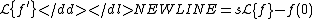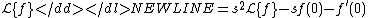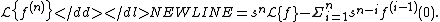Consider the following differential equation: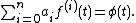This equation is equivalent to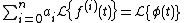which is equivalent to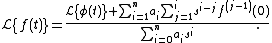Note that the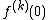are initial conditions.

The solution for f(t) will be given by applying the Laplace inverse transform to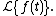## An example

We want to solve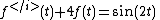with initial conditions f(0) = 0 and f ′(0)=0.

We note that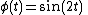and we get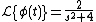So this is equivalent to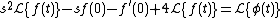We deduce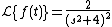So we apply the Laplace inverse transform and get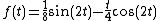The source of this article is wikipedia, the free encyclopedia.  The text of this article is licensed under the GFDL.